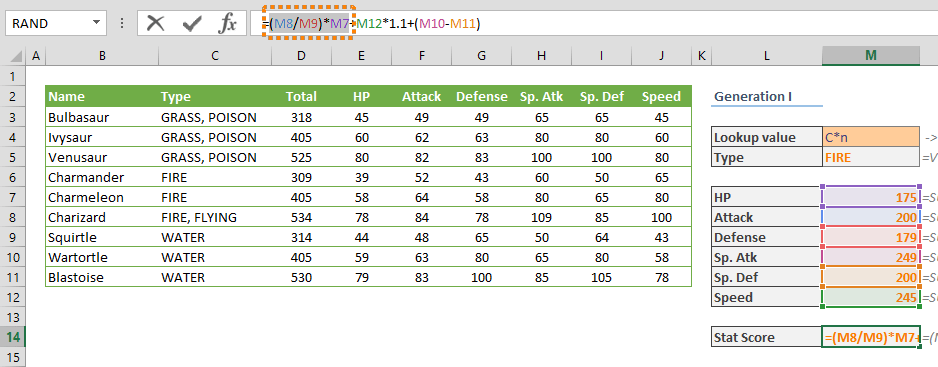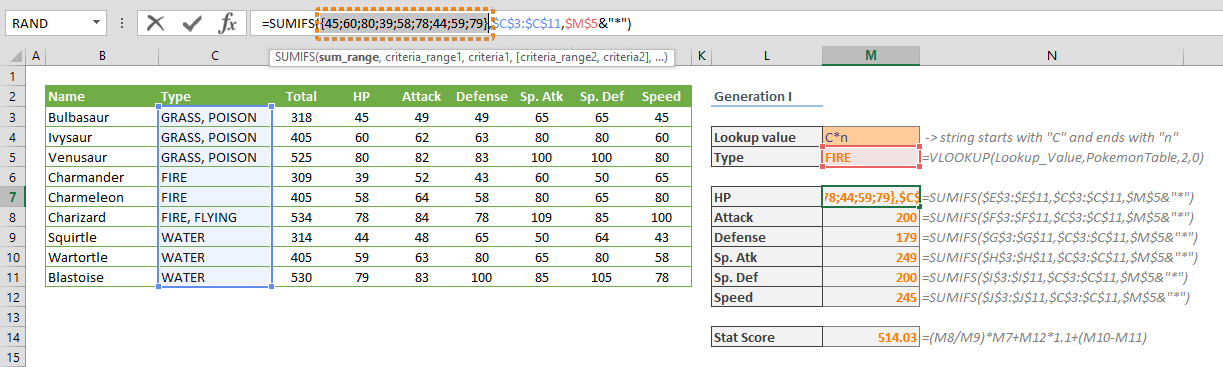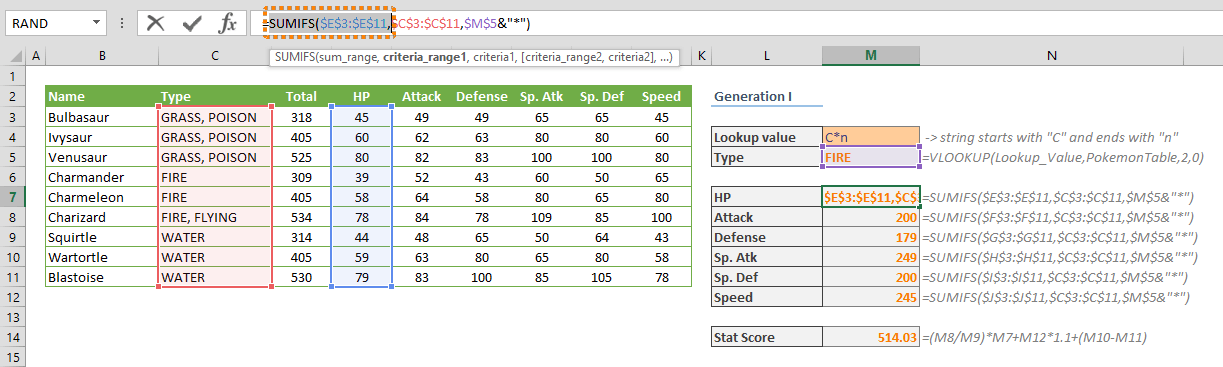Debugging formulas in Excel can be intimidating. The process becomes harder if you are trying to calculate and also remember the values of each part of the formula. Fortunately, the F9 key is an easy and quick way to break a formula down into pieces. The F9 key evaluates only the selected part of the formula. Thus, you can see the value that part stands in easily. In this guide, we're going to show you how to debug an excel formula using the F9 button and break down formulas.

Let's say, you have the following formula in cell M14:

=(M8/M9)*M7+M12*1.1+(M10-M11)

1. Select the cell (i.e. M14).
2. Press the F2 key to enter Edit mode, or activate this feature from the formula bar.3. Select the piece of the formula you want to evaluate.4. Press the F9 key. You will see the calculated value of the selection (i.e. (200/179)*175).This is essentially how to debug an Excel formula using the F9 key. However, there are few more things that you need to consider before using this feature.

## Remarks on debugging complex formulas with F9

1. Do not press the Enter key unless you intend to overwrite the formula part with the outcome value itself. Instead, press the Esc key to return to working on the spreadsheet.
2. Be careful when selecting a part of the formula. If you do not pay attention to the formula evaluating order, you may get incorrect results. For example, our sample formula returns approximately 03 with default values. However, if you select and evaluate (M8/M9), M7+M12 and 1.1+(M10-M11) parts, ignoring the priority of multiplication operation, you will get a value like 23510.61.

(200/179)*175+245*1.1+(249-200) = 514.03

=1.11731843575419*420*50.1 = 23510.61

1. if you evaluate a range reference you will get an array.2. You can't evaluate a part of the function. For example, the following scenario, a function name and its single argument are invalid. You should select all arguments.